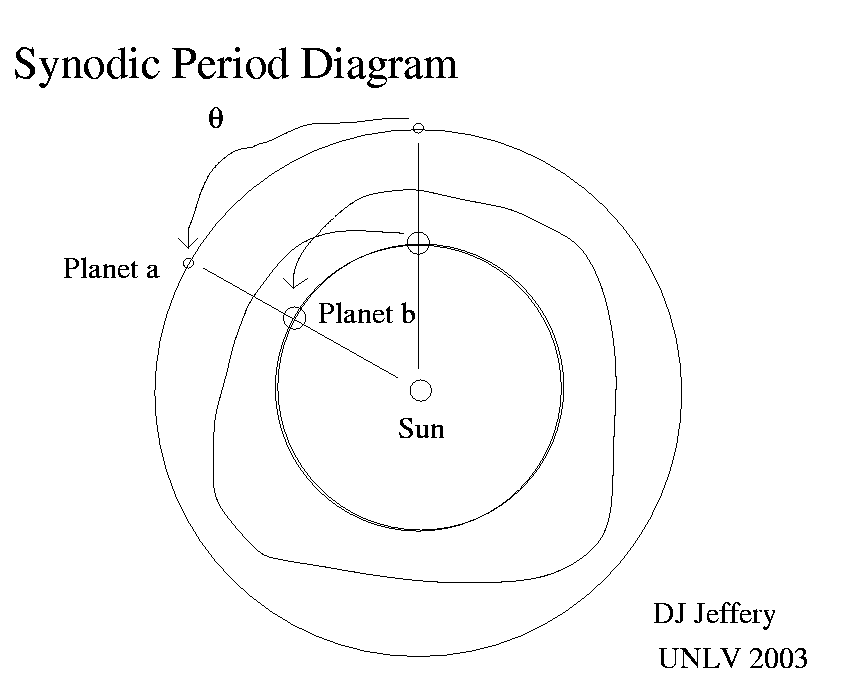# Synodic Period

1. A synodic period is the time it takes for an astro-body to return to the same angular position from the Sun as seen from the Earth. The term is usually used for the Moon and the planets. For the Moon the synodic period is the lunar month of 29.531 days on average. All synodic periods vary a bit, of course: everything in astronomy does.

2. We can show how to calculate approximate synodic periods for the planets easily. See the diagram below first.Planet b is an inner planet (an inferior planet relative to planet a in ancient astro jargon) and planet a is an outer planet (a superior planet relative to planet b in ancient astro jargon). Now planet b because it is inferior has a greater angular velocity about the Sun than planet a. Thus planet b will lap planet a eventually: i.e., planet b will travel from one point of alignment with planet a and the Sun (the vertical alignment in the diagram) and come into a new alignment (the oblique alignment in the diagram) after traveling more than 360 degrees. The time it takes to lap is the synodic period itself. If we measure angle from the vertical, then we have the following relations

360+\theta=r_a*t   and  \theta=r_b*t


where \theta is the angle of the second alignment, r_a is the angular speed of planet a, r_b is the angular speed of planet b, and t is the synodic period, our unknown. Now we solve for t eliminating \theta (which is an unknown that can stay unknown):

360+r_b*t=r_a*t,

t=360/(r_a-r_b), and thus

t=p_b*p_a/(p_b-p_a),


where p_a is the sidereal period of planet a and p_b is the sidereal period of planet b. Now in fact the synodic period of planet b relative to planet a is the same as that of planet a relative to planet b. Thus if we use absolute value signs, we need make no distinction in the formula between superior and inferior planets:

t=p_b*p_a/|p_b-p_a|.


If we assume p_a is constant, then we can see how t varies as p_b grows from 0 to p_a to infinity.

1. Intially, for p_b< When p_b goes to p_a, then t goes to infinity: the planets have the same period and are on the same orbit: one can never lap the other.
2. As p_b grows greater than p_a, t decreases from infinity and asymptotically approaches p_a: in the limit, planet b is practically standing still and the synodic period is just planet a's sidereal period.

3. Now the Moon's case is geometrically a little different than that of a planet, because the Moon orbits the Earth and the Earth orbits the Sun. But if you fix the Earth, you can regard the Sun and Moon as planets of the Earth: the Sun is superior and the Moon is inferior. With this picture, the algebra follows just as before and we get the same synodic period formula.

4. Synodic periods have great historical importance, because they allowed early astronomers to very easily calculate approximate planet ephemeris for some years in advance. For example, we can consider the Venus cycle that was known to the Babylonians by the 16th century BC at latest (No-28). The Venus synodic period is 583.92: 5 times this is 2919.6 days The Earth's year is approximately 365.25 days: 8 times this is 2922.0 days. Thus 2922.0 days or 8 full years is approximately equal to whole numbers of years and synodic periods. Thus, every 2922 days or 8 years Venus is the approximately returns to the same position relative to the Sun and the fixed stars. Thus one can compute where Venus will be today, just by looking up in a table where it was 8 years ago. Or you can draw up an ephemeris for Venus for 8 years ahead. By observing Venus for 8 years, you can predict its future day by day behavior indefinitely far into the future with increasing error, of course. But the error can be corrected 8 years in advance by continually Venus observation. It is a very simple procedure.

5. The Venus cycle is the easiest planet cycle, but others were developed for the other planets by the ancient Babylonians. One can imagine a cycle period in which all the planets return to the same positions on the sky. This period would have to be very long, of course. The ancient Greeks called this period the Great Year (No-39, No-167) and considered that it started when all the planets were aligned: i.e., they were all simultaneously in conjunction with the Sun. The astrologers wondered if history repeated itself every Great Year. I suppose there must be some huge number years that is approximately a common whole number multiple of all the planet synodic periods and the Earth's year, but a short web search doesn't show what it is. Anyway the ancient Babylonians, Greeks, and then Indians just used large mutiples of 360 years: they gave up trying to find a real Great Year. The Indian Mahayuga is 4,320,000 years or 1200*3600 years for example. (A yuga is some number of years: 2, 3, 4, 5, and 6 [No-163]. Maha I suppose means great as in maharaja.)